Ferromagnetism and Antiferromagnetism in 2D Hubbard model.

Hubbard Model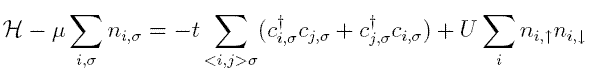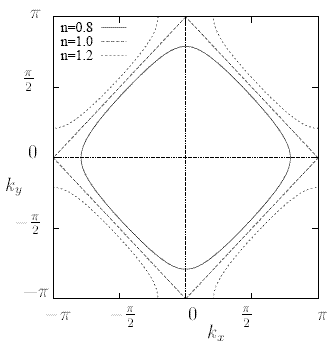Fig. 1: U=0 fermi surface of the Hubbard model for various fillings.

The Hubbard model, described by the Hamiltonian above, is a well known model to describe single s-bands in a tight-binding basis, with a local electron-electron repulsion U for electrons with opposite spin at the same atomic orbital. This model yields to collective effects such as itinerant magnetism and metal-insulator transition to name but a few.

Although simple in appearance, the model cannot be solved analytically except in one spatial dimension. In more than one spatial dimension, the model can be treated by several approximation techniques, among others mean field-theory, various Green's function decoupling schemes and functional methods.

U=0 Solution

In the simple case of U=0, and in a simple square lattice, the model has the following single particle eigenstates: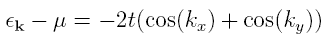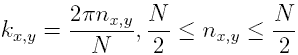N being the number of sites in x and y directions respectively and μ is the chemical potential ( μ=-U/2 in the case of half filling).

The Fermi surface in the half filled case has a very interesting and useful (as we will see later) feature called: "nesting". This means that a reciprocal lattice vector q=(π, ±π) maps an entire section of the Fermi surface onto another. In other words: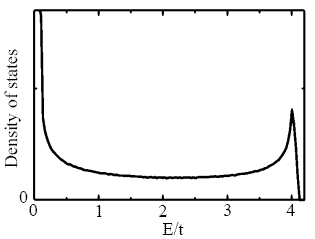Fig. 2: Hubbard model density of states at half-filling.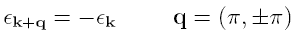Another useful feature of the model is the density of states for the non-interacting system, which is plotted below for the half filling case. A very interesting characteristic of the density of states, as shown in Figure 2 ( and calculated with the code, which can be found here ), is the singularity that appears in the middle (i.e the Fermi energy). This is the so called "Van Hove singularity". This singularity always occur in the two dimensional case and it is logarithmic with respect to the energy.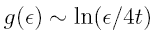The spin susceptibility per spin  is given by: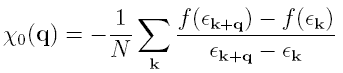It is important to notice in this case that the spin susceptibility diverges both the when q=0 and q= &pi . Responsible for the first divergence is the divergence of the density of states and for the second one, both the density of states divergence and the nesting of Fermi surface (always referring to half filling case).

Hartree-Fock and RPA for Nonzero U

RPA, or equivalently the sum of all the ladder diagrams , yields the following result for the spin susceptibility: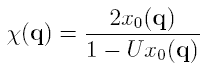Now we can discuss the Hartree-Fock (HF) approximation for this model. This is nothing but the following approximation for the Hamiltonian: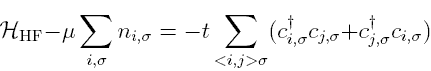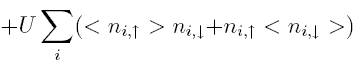This is nothing but one sort of mean field approximation to the Hubbard Hamiltonian. Now anticipating magnetic order we can assume (in terms of the filling n) for antiferromagnetic ordering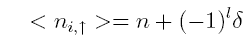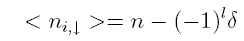and ferromagnetic ordering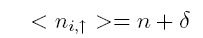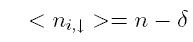respectively. Both of these two possible solutions yield the same gap equation: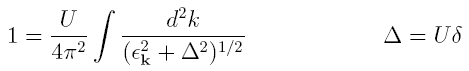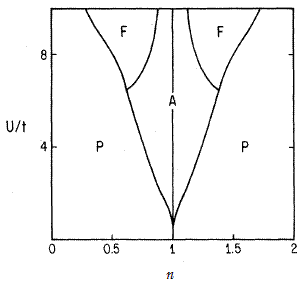Fig. 3: The regions of the Ferromagnetic, Antiferomagnetic and Paramagnetic ground states (in HF approximation) denoted by F,A and P respectively (calculated in ).

For half filling (n=1) the antiferromagnetic solution is the ground state and has a nonzero &delta for arbitrary U and the actual gap behaves like: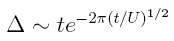We should comment on this result that it is due to both the divergence of the density of states at the Fermi energy and due to the "nesting" Fermi surface.

Now as far as the Ferromagnetic solution is concerned the situation is more complicated. First and foremost there is no Ferromagnetic ground state for half filling at any finite value of U/t. As we move away from half filling and we increase U/t though, Ferromagnetic ground states start to appear. There is also a famous theorem by Nagaoka  saying that for U=?, half filling and a single hole the Ferromagnetic solution has the lower energy. But the theorem has no extension to finite U/t and more than one hole and the issue is far from being solved.

Last but not least, there are some regions (of n and U/t) that the paramagnetic solution is the ground state, but this is just a limiting case of the other two types of magnetic solutions, with &delta=0.

© 2007 George Karakonstantakis. The author grants permission to copy, distribute and display this work in unaltered form, with attribution to the author, for noncommercial purposes only. All other rights, including commercial rights, are reserved to the author.

References

 J. E. Hirsch, Physical Review B 31, 4403 (1985).

 S. Doniac and E. Sondheimer, Green's Functions for Solid State Physicists (Benjamin, 1982), p. 162.

 Y. Nagaoka, Physical Review 147, 392 (1966).